Click to Chat

1800-1023-196

+91-120-4616500

CART 0

• 0

MY CART (5)

Use Coupon: CART20 and get 20% off on all online Study Material

ITEM
DETAILS
MRP
DISCOUNT
FINAL PRICE
Total Price: Rs.

There are no items in this cart.
Continue Shopping• Complete JEE Main/Advanced Course and Test Series
• OFFERED PRICE: Rs. 15,900
• View Details

```Revision Notes on Indefinite Integral

If the integrand is a derivative of a known function, then the corresponding indefinite integral can be directly evaluated.

If the integrand is not a derivative of a known function, the integral may be evaluated with the help of any of the following three rules:

1) Integration by substitution or by change of the independent variable.

2) Integration by parts

3) Integration by partial fractions

Some indefinite integrals which can be evaluated by direct substitutions:

1) If integral is of the form ∫ f(g(x)) g'(x) dx, then put g(x) = t, provided ∫ f(t) exists.

2) ∫ f'(x)/f(x) dx = ln |f (x)| + c,  By putting f (x) = t => f' (x) dx = dt

=> ∫ dt/t = ln |t| + c = ln |f (x)| + c.

3) ∫ f'(x)√f(x) dx = 2 √f(x)+c,  Put f (x) = t

Then ∫ dt/√t = 2√t + c = 2√f(x) + c.

Some standard substitutions:

1) For terms of the form x2 + a2 or √x2 + a2, put x = a tanθ or a cotθ

2) For terms of the form x2 - a2 or √x2 – a2 , put x = a sec θ or a cosecθ

3) For terms of the form a2 - x2 or √x2 + a2, put x = a sin θ or a cosθ

4) If both √a+x, √a–x, are present, then put x = a cos θ.

5) For the form √(x–a)(b–x), put x = a cos2θ + b sin2θ

6) For the type (√x2+a2±x)n or (x±√x2–a2)n, put the expression within the bracket = t.

7) For 1/(x+a)n1 (x+b)n2, where n1,n2 ∈ N (and > 1), again put (x + a) = t (x + b)

If the integrand is of the form f(x)g(x), where g(x) is a function of the integral of f(x), then put integral of f(x) = t.

The integral of product of two functions of x is evaluated with the help of integration by parts. Let u and v be two functions of x, then ∫uv dx = u∫v dx - ∫[du/dx ∫v dx]dx

While carrying out integration by parts, whether a function is u or v should be decided according to ILATEmethod of integration (Inverse, Logarithmic, Algebraic, Trigonometric, Exponent).

If both the functions are directly integrable then the first function is chosen in such a way that the derivative of the function thus obtained under integral sign is easily integrable.

If in the product of the two functions, one of the functions is not directly integrable like lnx, sin-1x, cos-1x, tan-1x etc. then we take it as the first function and the remaining function is taken as the second function.

If there is no second function available, then unity is taken as the second function e.g. in the integration of∫tan-1x dx, tan-1x is taken as the first function and 1 as the second function.

In the integral ∫g(x)exdx, if g(x) can be expressed as g(x) = f(x) + f'(x) then ∫g(x)ex dx=∫ ex [f(x) + f'(x)] dx = exf(x) + c

To write P(x)/ Q(x) in partial fractions, write Q(x) in the form Q(x) = (x – a)k ... (x2 + αx + β)r ... where binomials are different, and then set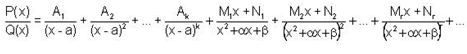Where A1, A2, ...,Ak, M1, M2, ......, Mr, N1, N2, ...... ,Nr are real constants to be determined.

A rational function P(x)/Q(x) is proper if the degree of polynomial Q(x) is greater than the degree of the polynomial P(x).

If the degree of P(x) is greater than or equal to the degree of Q(x), we first write P(x)/Q(x) = h(x) + P(x)/Q(x), where h(x) is a polynomial and p(x) is a polynomial of degree less than the degree of polynomial Q(x).

In integrals of the form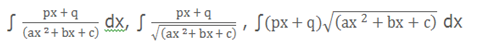1) Substitute px + q = l (which is the differential coefficient of ax2 + bx + c) + m.

2) Find l and m by comparing the coefficient of x and constant term on both sides of the identity.

3) The question will then reduce to the sum of two integrals which can be integrated easily.

In case of integrals of the type1) Substitute ax2 + bx + c = M (px2 + qx + r) + N (2px + q) + R.

2) Find M, N, & R.

3) The integration reduces to integration of three independent functions.

Irrational functions of the form (ax+b)1/nand x can be easily evaluated by the substitutiontn = ax+ b. Thus ∫f(x, (ax + b)1/n)dx = ∫ f ((tn – b)/ a , t) ntn-1 / a dt

In case of integrals of the form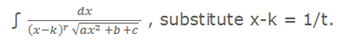In order to compute the indefinite integrals of the form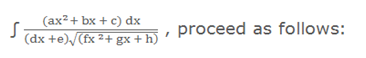1) Write, ax2 +bx+c  = A1 (dx +e) ( 2fx +g) +B1( dx +e) +C1where A1, B1 and C1 are constants which can be obtained by comparing the coefficient of  like terms on both sides.

2) The given integral will then reduce to the form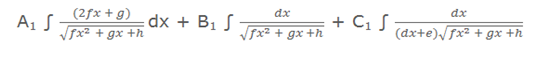Integrals of the form ∫ R (sin x, cos x) dx can be solved by substituting tan(x/2) = t. Hence, the rest of the trigonometric functions can be substituted as sin x = 2t/(1+t2) , cos x = (1-t2)/(1+t2) , x = 2 tan-1t and so dx = dt/(1+t2)

Majority of the integrals of the form ∫ R (sin x, cos x) dx can be solved by the above method, but in some cases the substitution cot x/2 = t may prove useful. Some other substitutions that can be used in specific cases include:

1) If R(-sin x, cos x) = -R(sin x, cos x), substitute cos x = t

2) If R(sin x, -cos x) = -R(sin x, cos x), substitute sin x = t

3) If R(-sin x, - cos x) = R(sin x, cos x), substitute tan x = t

For integrals of the form ∫ (pcosx + q sinx + r) / (a cos x + b sin x + c) dx:

1) Express the numerator as m (denominator) + l (differential coefficient of denominator) + n.

2) Now, compute m, l and n by comparing the coefficients of sin x, cos x and constant term and split the integral into sum of three integrals.

3) This can be expressed as l∫ dx + m ∫d.c. of (Denominator) / denominator dx + n ∫ dx/ (a cos x + b sin x + c)

Integrals of the form ∫ (pcos x + q sin x) / (a cos x + b sin x) dx can be solved by expressing the numerator as l (denominator) + m(d.c. of denominator) and then find l and m as discussed above.

In case of integrals of the type ∫(sinmx cosnx)dx, where m, n ∈ natural numbers, the following substitutions prove to be helpful:

1) If one of them is odd, then substitute for term of even power.

2) If both are odd, substitute either of the term.

3) If both are even, use trigonometric identities only.

The various indefinite integral formulae which must be remembered as they are extremely helpful in solving problems include:

1) ∫ exdx = ex + c

2) ∫ 1/x dx = ln |x| + c

3) ∫ ax dx = ax/ ln a + c  (a > 0)

4) ∫ cos x dx = sin x + c

5) ∫ sin x dx = - cos x + c

6) ∫ sec2 x dx = tan x + c

7) ∫ cosec x cot x dx = - cosec x + c

8) ∫sec x tan x dx = sec x + c

9) ∫ cosec2 x dx = - cot x + cIntegration by parts:

This method is used to integrate the product of two functions. If f(x) and g(x) are two integrable functions, then

∫f(x) g(x) dx = f(x) ∫ g(x) dx – ∫ {d/dx (f(x)) . ∫g(x) dx} dx.

In order to select the first function, the following order is followed:

Inverse → Logarithmic → Algebraic → Trigonometric → Exponential

Integration using partial fractions:

If f(x) and g(x) are two polynomials, and deg (f(x)) < deg (g(x)), then f(x)/g(x) is called a proper rational fraction.

If deg (f(x)) ≥ deg (g(x)), then f(x)/g(x) is called an improper rational fraction.

If f(x)/g(x) is an improper rational function, then divide f(x) by g(x) and convert it to proper rational function i.e. f(x) / g(x) = l(x) + h(x)/g(x).

Any proper rational function f(x)/g(x) can be expressed as teh sum of rational functions each having a factor of g(x). Each of these factors is called a partial fraction and the process of obtaining them is called decomposition of f(x)./g(x) into partial fractions.
```### Course Features

• 728 Video Lectures
• Revision Notes
• Previous Year Papers
• Mind Map
• Study Planner
• NCERT Solutions
• Discussion Forum
• Test paper with Video Solution